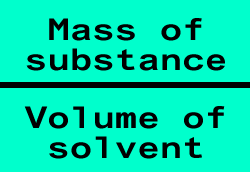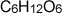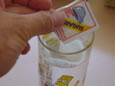Concentration mass/volume The amount of substance that is dissolved in a certain volume of water, or any other solvent, is known as the concentration. Concentration is very important to us, we use it to measure the safe levels of heavy metals (pollutants) in our food, to administer medication and for countless other functions. Concentration can be expressed in many forms, grams per litre, parts per billion, parts per million, moles per litre, just to name a few.Example. 18 grams of sugar() was poured into a cup containing 100ml of water. What is the concentration of the sugar solution in a)grams per ml? b) mole per litre?Solution a) Step 1 What is the mass of the sugar? = 18 grams Step 2 How many mls of solvent are present?=100mls Step 3 Concentration is amount/volume = 18g/100mls = 0.18 grams per ml Solution b) Step 1 Convert the mass of the sugar into moles of sugar (as we have discussed in previous exercises) mass/formula mass = mole, 18/180=0.1mole of sugar Step 2 How many litres of solvent are present? = 100mls/1000mls =0.1litres Step 3 Concentration is amount/volume = 0.1/0.1 =1mole per litre.

Home